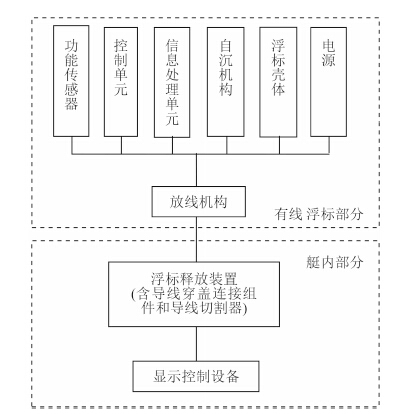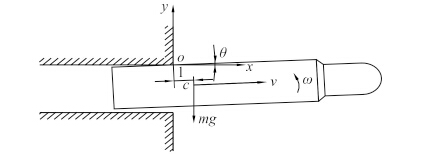﻿ 潜用抛弃式有线浮标及其关键技术
 舰船科学技术2017, Vol. 39Issue (2): 137-140PDF

Submarine wired disposable buoy and key techniques
LIU Rui-hai, XU Wen-tao, ZHANG Liang
China Ship Development and Design Center, Wuhan 430064, China
Abstract: Submarine wired disposable buoy (SWDB) could be deployed to the surface to receive information by submerged submarine. In this paper, the function and components of SWDB are introduced; the key techniques and solution considerations are also presented.
Key words: wired disposable buoy     submarine     technique

1 浮标功能及组成图 1 有线浮标系统组成示意图 Fig. 1 SWDB system simplified diagram
2 关键技术及其解决思路 2.1 有线浮标总体设计技术

2.2 导线动态安全释放技术

2.3 有线浮标发射适配技术

2.4 浮标离管安全控制技术图 2 浮标出管运动示意图 Fig. 2 SWDB initial mechanical analysis of launching
 $\left\{ \begin{array}{l} x = l\cos \theta+r\sin \theta\text{，} \\ y = l\sin \theta - r\cos \theta \text{。} \end{array} \right.$ (1)

 $\left\{ \begin{array}{l} {F_l} = ({F_y}+mg)(\sin \theta - r'\cos \theta )+({F_x}+P)(\cos \theta+r'\sin \theta )\text{，}\\[5pt] {F_\theta } = ({F_v}+mg)(l\cos \theta - r\sin \theta )+({F_x}+P)(r\cos \theta - l\sin \theta )\text{。} \end{array} \right.$ (2)

 $\frac{\rm d}{{{\rm d}t}}\left[ {\frac{{\partial T}}{{\partial q}}} \right] - \frac{{\partial T}}{{\partial q}} = {F_q}\text{。}$ (3)

 $\left\{ \begin{array}{l} \frac{\rm d}{{{\rm d}t}}\left[ {(1+{{r'}^2})\frac{{{\rm d}l}}{{{\rm d}t}}+(r - r'l)\frac{{{\rm d}\theta }}{{{\rm d}t}}} \right] - (l+rr'){\left[ {\frac{{{\rm d}\theta }}{{{\rm d}t}}} \right]^2} = \frac{{{F_l}}}{m}\text{，}\\[5pt] \frac{\rm d}{{{\rm d}t}}\left[ {({l^2}+{r^2}+{k^2})\frac{{{\rm d}\theta }}{{{\rm d}t}}+(r - r'l)\frac{{{\rm d}l}}{{{\rm d}t}}} \right] = \frac{{{F_\theta }}}{m}\text{。} \end{array} \right.$ (4)

 $\left\{ \begin{array}{l} {\rm d}l/{\rm d}t = {v_l}\text{，}\\ {\rm d}\theta /{\rm d}t = \omega \text{。} \end{array} \right.$ (5)

2.5 浮标在海面的姿态稳定技术

3 国外应用情况

21 世纪初，英美两国又联合开展了潜用可回收光纤系留浮标系统（RTOF）的研制。该系统的系留浮标体相对较大，尾部带有一根光缆与绞车装置连接。浮标体从装在潜艇上的存储舱中放出后，靠自身浮力漂浮至水面，同时潜艇上的绞车装置不断释放光缆使其几乎不受拉力。该浮标体可搭载通信、导航、侦察等功能部件，浮标体到达水面后漂浮在海面执行相应任务，而潜艇继续根据航行速度不断布放光缆以避免产生大的拉力，保持浮标体在海面的位置基本不变；在任务完成后，绞车反转，拖动浮标体收回到潜艇。

4 结 语

  严巍. 两种新型潜艇通信浮标研究概况[J]. 舰船电子工程, 2006 (4): 31–34. YAN Wei. Research on two new types of submarine communication buoy[J]. Ship Electronic Engineering, 2006 (4): 31–34.  史伟, 野学范, 孙晓磊. 多功能抛弃式浮标及在潜艇作战中的运用[J]. 四川兵工学报, 2011 (6): 150–152.  董晓明, 刘慷. 线导鱼雷及其关键技术[J]. 现代军事, 2001 (12): 39–41.  肖尚彬, 董秋泉. 高等动力学[M]. 西安: 西北工业大学出版社, 1986.  秦锋, 逄洪照. 基于第二类拉格朗日方程的水雷内末弹道仿真[J]. 鱼雷技术, 2008 (5): 12–14. QIN Feng, PANG Hong-zhao. Simulation of interior trajectory terminal for mine based on second lagrange formulation[J]. Torpedo Technique, 2008 (5): 12–14.  王光源, 李冬, 李伟波. 抛弃式通信浮标对潜艇隐蔽性的影响[J]. 舰船科学技术, 2011, 33 (10): 112–115. WANG Guang-yuan, LI Dong, LI Wei-bo. Research on the impact on disguise of submarine with disposable communication buoy[J]. Ship Science and Technology, 2011, 33 (10): 112–115.  赵登平. 潜艇信息传输[J]. 舰船知识, 2014 (11): 37–41.  孙东平, 荣海洋, 张靖康. 卫星浮标天线技术及其在潜艇通信中的应用[J]. 装备环境工程, 2009 (5): 54–56. SUN Dong-ping, RONG Hai-yang, ZHANG Jing-kang. Satellite buoy antenna technology and its application in submarine communication[J]. Equipment Environmental Engineering, 2009 (5): 54–56.